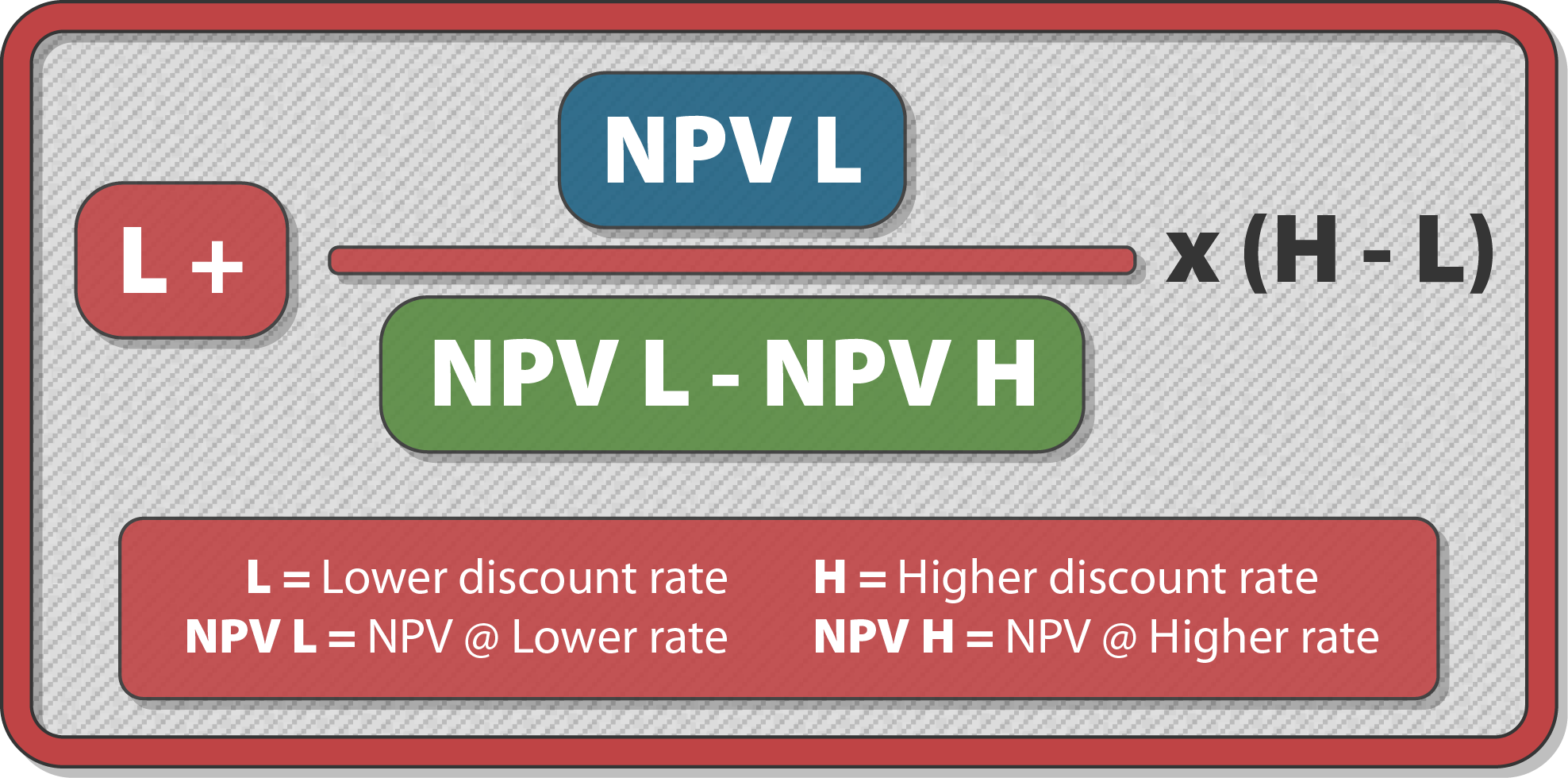MAF2
Syllabus D. Budgeting D4. Capital Budgeting and Discounted Cash Flows

# ACCA MA F2 Syllabus D. Budgeting - D4h. Net present value (NPV) and Internal rate of return (IRR) - Notes8 / 10

### Syllabus D4h)

Explain and illustrate the net present value (NPV) and internal rate of return (IRR) methods of discounted cash flow

### Discounted Cash Flow

Discounted cash flow, or DCF, is an investment appraisal technique that takes into account both the timing of cash flows and also the total cash flows over a project’s life.

#### Net Present Value

The NPV is the value obtained by discounting all the cash outflows and inflows for the project capital at the cost of capital and adding them up.

Hence, it is the sum of the present value of all the cash inflows from a project minus the PV of all the cash outflows.

NPV is positive – the cash inflows from a capital investment will yield a return in excess of the cost of capital.

The project is financially attractive

NPV is negative – the cash inflows from a capital investment will yield a return below the cost of capital.

From a financial perspective, the project is therefore unattractive.

NPV is exactly zero - the cash inflows from a capital investment will yield a return exactly equal to the cost of capital.

The project is therefore just about financially attractive.

If a company has 2 projects under consideration it should choose the one with the highest NPV.

#### Illustration

Initial investment (\$1,000)

Inflows:
Year 1 \$900
Year 2 \$800
Year 3 \$700

The cost of capital is 10%.

What is the net present value?

Solution

Year 1 \$900 x 0.909 = \$818
+ Year 2 \$800 x 0.826 = \$661
+ Year 3 \$700 x 0.751 = \$526

(\$1,000)

= \$1,005 is the NPV

#### Internal Rate of Return

The internal rate of return (IRR) is essentially the discount rate where the initial cash out (the investment) is equal to the PV of the cash in.

So, it is the discount rate where the NPV = 0.

If the IRR is higher than a target rate of return, the project is financially worth undertaking.

Consequently, to work out the IRR we need to do trial and error NPV calculations, using different discount rates, to try and find the discount rate where the NPV = 0.

This is known as the interpolation method.

The steps in this method are:

1. Step 1: Calculate two NPV for the project at two different costs of capital. It is important to find two costs of capital for which the NPV is close to 0, because the IRR will be a value close to them.

2. Step 2: Having found two costs of capital where the NPV is close to 0, we can then estimate the cost of capital at which the NPV is 0, i.e. the IRR.

A formula is used:#### Illustration

At 12% cost of capital, the NPV was \$10,000
At 18% cost of capital, the NPV was (\$1,000)

What is the IRR?

Solution

12%+(10,000/10,000- - 1,000)(18%-12%)

= 17.5% is the IRR

#### Mutually Exclusive Investments

The rule for deciding between mutually exclusive projects is to accept the project with the higher NPV.